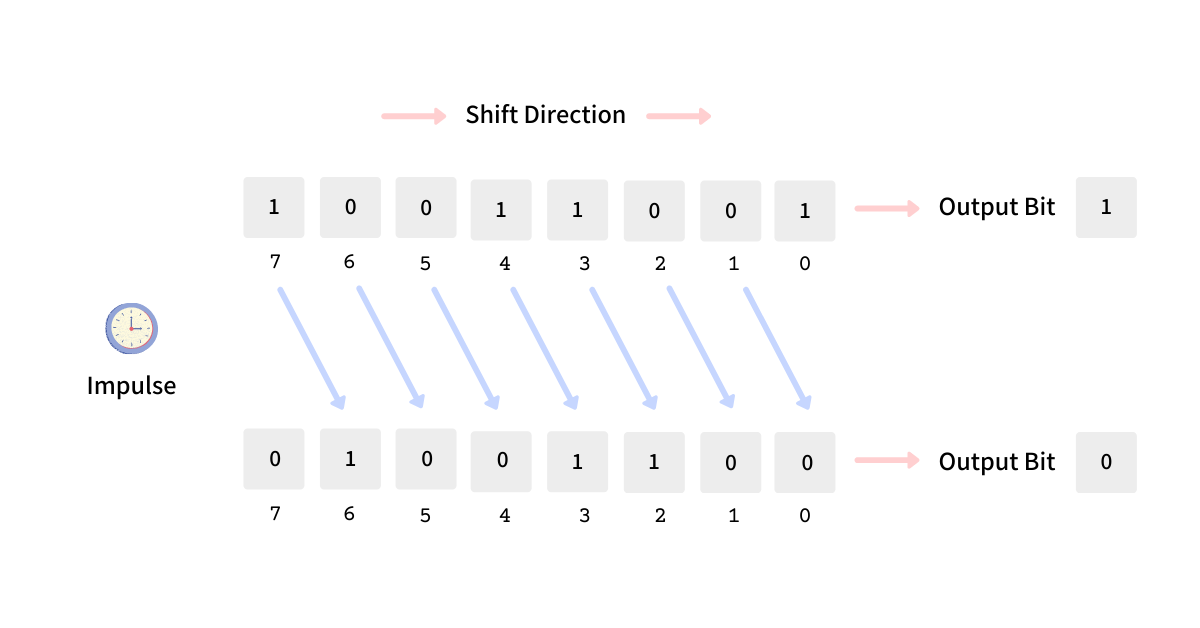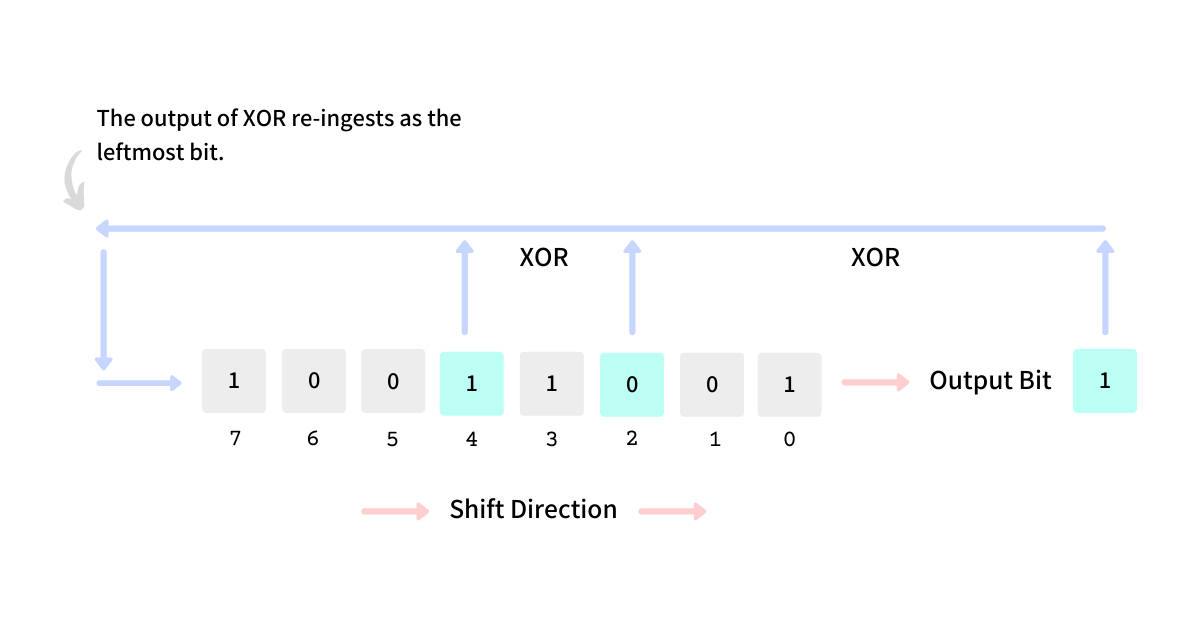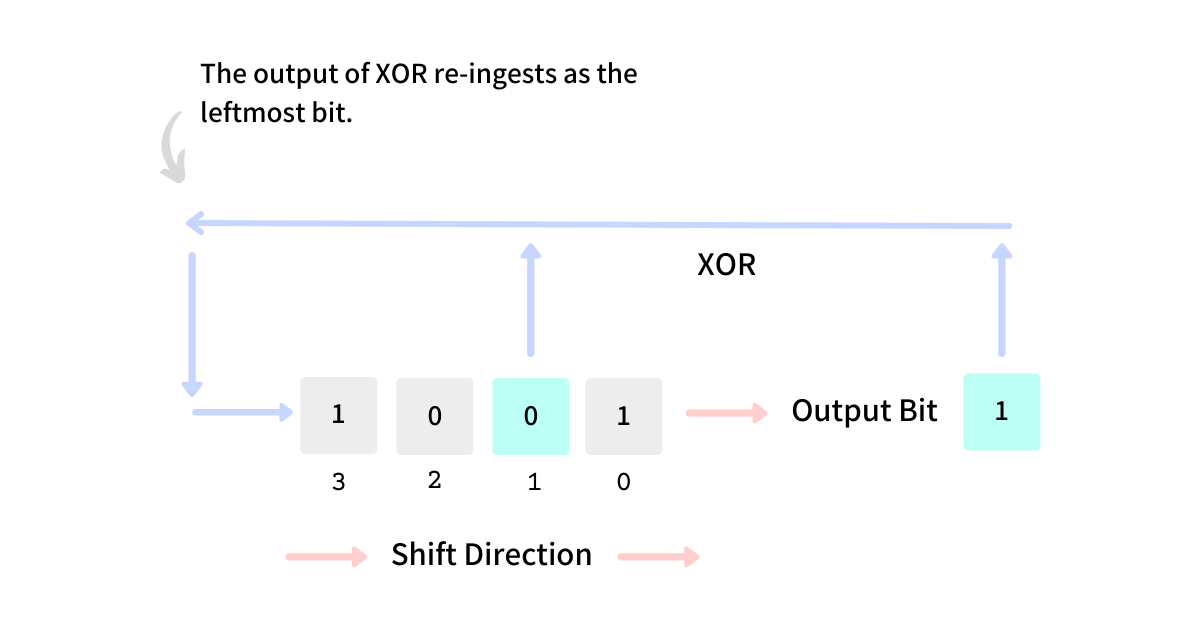# Simplest algorithm to generate a pseudorandom number

A few essays back, we saw how pseudorandom numbers are generated using Cellular Automaton - Rule 30. This essay takes a detailed look into random number generation using LFSR - Linear Feedback Shift Registers. LFSR is widely adopted to generate random numbers on microcontrollers because they are very simple, efficient, and easy to adopt both hardware and software.

# Pseudorandom Number Generators

A pseudorandom number generator produces numbers that seem aperiodic (random) but are deterministic. It uses a seed value and generates a sequence of random numbers as a function of the current state and some previous states.

These are pseudorandom (not truly random) because the following numbers can be determined algorithmically if the seed value is known. True random numbers are either generated using hardware or from natural phenomena like blood volume pulse, atmospheric pressure, thermal noise, quantum phenomenon, etc.

# What is LFSR

A linear feedback shift register is a collection of bits that shifts when triggered, and the next state is a linear function of its previous state. We use right-shift (`>>`) as the shift operation and XOR (`^`) as the linear function to generate the next state of the register.The LFSR is initiated with a random value called a seed. The next state of the register can be computed deterministically using its previous state and the mentioned operations.

# LFSR in action

A series of latches (bits) connected to the next in line forming a chain is called a register. The diagram below shows an 8-bit shift register that shifts to the right upon an impulse. The rightmost bit that is thrown out during the shift is the output bit.When the shift happens, the leftmost latch gets vacant, and it is either

• filled with the output bit forming a circular shift register
• filled with zero, like a pure right shift operation of a programming language
• filled with a result of some boolean logic on the latches

LFSR that we used to generate pseudorandom numbers goes with the third approach and applies a boolean XOR on a set of chosen latches, called taps, and puts the resultant bit in the leftmost latch, creating a Linear Feedback.

## A simple 4-bit LFSR

An LFSR has 3 configurable parameters

• number of bits in the register - `n`
• initial n-bit seed value - `seed`
• position of taps for XOR - `taps`

We build a simple `4`-bit LFSR with a seed value of `0b1001` and tap position of `1`. The output bit will be the rightmost bit of the register, and the next state of the LFSR will be computed as the

• XOR the output bit with the bit in the `1`st position (indexed at 0) from the right
• shift the bits of the register by one to the right
• set the vacant leftmost bit with the output of the XOR operationAfter all the above operations are completed, the set of bits in the LFSR becomes the current state and is then used to output the next random bit, thus continuing the cycle.

The above example is taken from the Computerphile’s video on this same topic.

Golang-based implementation of the above LFSR is as shown below. We define a struct holding LFSR with the mentioned three parameters - the number of bits in the register, the seed, and the position of the taps. We define the function named `NextBit` on it that returns a pseudorandom bit generated with the logic mentioned above.

``````type LFSR struct {
n    uint32
seed uint32
taps []uint32
}

func (l *LFSR) NextBit() uint32 {
seed := l.seed

// output bit is the rightmost bit
outputBit := l.seed & 1

// XOR all the bits present in the tap positions
for _, tap := range l.taps {
seed = seed ^ (l.seed >> tap)
}

// the new msb is the output of this XOR
msb := seed & 1

// rightsift the entire seed
// and place newly computed msb at
// the leftmost end
l.seed = (l.seed >> 1) | (msb << (l.n - 1))

// return the output bit as the next random bit
return outputBit
}``````

When we execute the above code with seed `0b1001`, tap position `1`, on a `4`-bit LFSR, we get the following random bits as the output, and with a little bit of pretty-printing, we see

``````lfsr: 1001       output: 1
lfsr: 1100       output: 0
lfsr: 0110       output: 0
lfsr: 1011       output: 1
lfsr: 0101       output: 1
lfsr: 1010       output: 0
lfsr: 1101       output: 1
lfsr: 1110       output: 0
lfsr: 1111       output: 1
lfsr: 0111       output: 1
lfsr: 0011       output: 1
lfsr: 0001       output: 1
lfsr: 1000       output: 0
lfsr: 0100       output: 0
lfsr: 0010       output: 0
lfsr: 1001       output: 1
lfsr: 1100       output: 0
lfsr: 0110       output: 0
lfsr: 1011       output: 1
lfsr: 0101       output: 1``````

The output bits seem random enough, but upon a close inspection, we see that the last 5 output bits are the same as the first 5, and it is because, for the given configuration of seed and tap, the value in the LFSR becomes the same as the seed value `0b1001` after 15 iterations; thus, from the 16th position, we can see the same set of output bits generated.

By carefully selecting the seed and the taps, we can ensure that the cycle is long enough to never repeat in our process’s lifetime. You can find the detailed source code for this LFSR at github.com/arpitbbhayani/lfsr.

## LFSR Bits to Number

Although LFSR generates pseudorandom bits, generating random numbers is fairly simple using it. To generate a `k`-bit random number we need to generate `k` random bits using our routine LFSR and accumulate them in a `k`-bit integer, which becomes our random number.

Golang-based implementation of the above logic can be seen in the function named `NextNumber` defined below.

``````func (l *LFSR) NextNumber(k int) uint32 {
var n uint32 = 0

// generate a random bit using LFSR
// set the random bit in the lsb of a number
// left-shift the number by 1
// repeat the flow `k` times for a k-bit number
for i := 0; i < k; i++ {
n = n << 1
n |= l.NextBit()
}
return n
}``````

The first ten 8-bit random numbers generated with a seed `0b1001` and tap position `1` using the above logic are

``````154
241
53
226
107
196
215
137
175
19``````

We can see that the numbers are fairly random and are within the limits of an 8-bit integer. You can find the detailed source code for this LFSR at github.com/arpitbbhayani/lfsr.

# Applications of LFSR

LFSRs find their application across a wide spectrum of use cases, given how efficient they generate randomness. Their applications include digital counters, generating pseudorandom numbers, pseudo-noise, scramble radio frequencies, and in general, a stream of bytes. We take a detailed look into LFSR for scrambling.

## Scrambling using LFSR

LFSRs are computationally efficient and deterministic for a seed value, i.e., they generate the same set of numbers in the same order for a seed value, and here’s how they find their application in scrambling and unscrambling a stream of bytes.

The idea of scrambling using LFSR goes like this. We read the raw bytes from the file and pass them to the scrambler function. The function initializes the LFSR with the necessary register length, seed, and tap positions and is kept ready to generate the 8-bit random numbers.

The stream of bytes, from the input file, is then XORed with the random numbers generated from the LFSR such the `i`th byte of the file is XORed with the `i`th 8-bit number generated from the LFSR. Golang-based implementation of `scramble` function is as shown below.

``````func scramble(in []byte, n uint32, seed uint32,
taps []uint32) []byte {

// initiatializing LFSR with the provided config
l := lfsr.NewLFSR(n, seed, taps)

// creating an output byte slice
out := make([]byte, len(in))

// XOR byte by byte
for i := range in {
out[i] = in[i] ^ byte(l.NextNumber(8))
}

// return the output slice
return out
}``````

The unscrambling process exploits the following property of XOR and the fact that the LFSR will generate the same set of random numbers in the same order for the given configuration.

``a XOR b XOR a = b``

So, if we XOR a byte twice with the same number, we get the same byte in return. We apply the same `scramble` function even to unscramble the scrambled data.

``````func unscramble(in []byte, n uint32, seed uint32,
taps []uint32) []byte {

// invoking the same scramble function
return scramble(in, n, seed, taps)
}``````

# Concerns with LFSR

Although LFSRs are very efficient both on the software and hardware sides, there are some concerns about using them.

The number of bits in the LFSR is limited (as configured); the register will repeat the same set after generating a certain set of bits. The length of the cycle depends solely on the seed value and the tap configuration. So, while employing LFSR for random number generation, it is essential to pick a good set of tap positions and seeds to ensure a very long cycle.

Suppose we get hold of a few consecutive random numbers generated from the LFSR. In that case, we can put them in a few linear equations and reach the initial configuration, enabling us to predict the future set of random numbers. Given how vulnerable LFSRs can be, they are not used at places that need cryptographic strength.

### Courses

Super practical courses, with a no-nonsense approach, are designed to spark engineering curiosity and help you ace your career.

## System Design for Beginners

An in-depth, self-paced, and on-demand course that for early engineers to become great at designing scalable, available, and extensible systems at scale.

## System Design Masterclass

A masterclass that helps experienced engineers become great at designing scalable, fault-tolerant, and highly available systems.

## Redis Internals

A course that helps covers Redis internals by reimplementing its core features like - event loop, serialization protocol, pipelining, eviction, and transactions.## CS newsletter for the curious engineers

If you like what you read subscribe you can always subscribe to my newsletter and get the post delivered straight to your inbox. I write essays on various engineering topics and share it through my weekly newsletter.

Writings and Videos
Legal and Contact
Everything Else